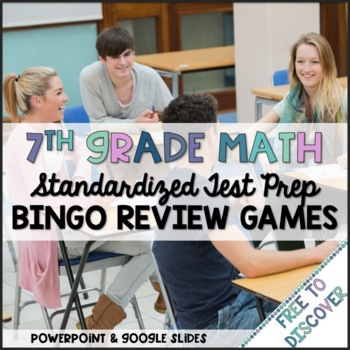digital

# 7th Grade Math Review Games Bundle | Distance LearningSubject
Resource Type
Format
Zip (20 MB)Google Apps™
\$12.00
Bundle
List Price:
\$16.25
You Save:
\$4.25
\$12.00
Bundle
List Price:
\$16.25
You Save:
\$4.25This bundle contains one or more resources with Google apps (eg. docs, slides, etc.).

#### Also included in

1. This seventh grade math MEGA (growing) bundle is on its way to becoming a complete 7th grade math curriculum. This year I am adding differentiated notes and practice for 10 units to form a complete 7th grade math curriculum. It currently contains all discovery worksheets, card games, task cards, s
\$210.00
\$320.75
Save \$110.75

### Description

These PowerPoint BINGO review activities are aligned to all 7th grade Common Core standards. They have been divided up into the five strands: Ratios and Proportions, The Number System, Expressions & Equations, Geometry, and Statistics and Probability. Perfect for standardized test prep, end of year 7th grade review, or beginning of the year 8th grade review.

~~~~~~~~~~~~~~~~~~~~~~~~~~~~~~~~~~~~~~~~

Students work in cooperative groups to review how to:

-compute unit rates involving fractions.

-compute unit rates found within real world problems.

-determine whether a relationship is proportional.

-identify the constant of proportionality given a table or graph.

-represent proportional relationships by equations.

-describe the meaning of a point on a graph.

-calculate percent problems involving tax, discount, markups, and simple interest.

-add, subtract, multiply, and divide fractions.

-add, subtract, multiply, and divide decimals.

-add, subtract, multiply, and divide integers.

-identify the meaning of integers in real scenarios.

-combine like terms.

-distribute and factor.

-rewrite an expression in different forms.

-evaluate expressions involving rational numbers.

-solve real world problems involving rational numbers.

-solve two-step equations and inequalities.

-solve word problems involving two-step equations and inequalities.

-solve problems involving scale drawings of geometric figures.

-draw geometric shapes given conditions.

-identify two-dimensional shapes that result from slicing a three-dimensional figure.

-find area and circumference of circles.

-write and solve basic equations based on angle relationships.

-determine area of composite two-dimensional figures.

-determine volume and surface area of three-dimensional prisms.

-identify bias in certain sampling methods.

-understand that random sampling results in a representative sample and can be used to make predictions and/or generalizations about the population.

-determine measures of center and use the information to compare data sets.

-determine the mean absolute deviation of data sets and use this data to compare.

-construct and interpret line plots to compare data sets.

-identify whether the probability is likely, unlikely, or neither.

-understand the relationship between experimental probability and theoretical probability.

-calculate simple probability.

-calculate compound probability.

-determine the number of outcomes using an organized list and tree diagram.

~~~~~~~~~~~~~~~~~~~~~~~~~~~~~~~~~~~~~~~~

Included in this product:

-5 PPT games each with 18 review questions aligned to the CCSS (with answers)

-BINGO board

-Number Cards 1-24 (directions for use included)

-Teacher's Guides

**************************************************************************

☺ Earn TpT credits to use toward future purchases by providing feedback for paid products. (Feedback on free products is also greatly appreciated!)

☺ Follow me to receive notifications when new products are posted. This will help you secure the 50% discount available for the first 48 hours. You will also receive occasional messages alerting you to sales, promotions, and other exciting news.

☺ Don’t quite see what you are looking for? Leave me a note in the “Ask a Question” tab. I would be happy to create a product to meet the needs of you and your students.

The purchase of this product entitles a single user to reproduce the resource for classroom use only. Discounted additional licenses can be purchased if you wish to share with other teachers. The product is for educational use only. The product may not be used for commercial purposes or resold in any form. It cannot be uploaded to the Internet, with the exception of password-protected school websites.

© Free to Discover (Amanda Nix)

Total Pages
N/A
Included
Teaching Duration
1 Week
Report this Resource to TpT
Reported resources will be reviewed by our team. Report this resource to let us know if this resource violates TpT’s content guidelines.